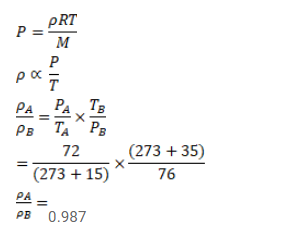# Use R=8.3 J/mol-K wherever required.Question:

Use $\mathrm{R}=8.3 \mathrm{~J} / \mathrm{mol}-\mathrm{K}$ wherever required.

The temperature and pressure at Shimla are $15.0^{\circ} \mathrm{C}$ and $72.0 \mathrm{~cm}$ of mercury and at Kalka these are $35.0^{\circ} \mathrm{C}$ and $76.0 \mathrm{~cm}$ of mercury. Find the ratio of air density at Kalka to the air density at Shimla.

Solution: